+关注继续查看

# 以下为译文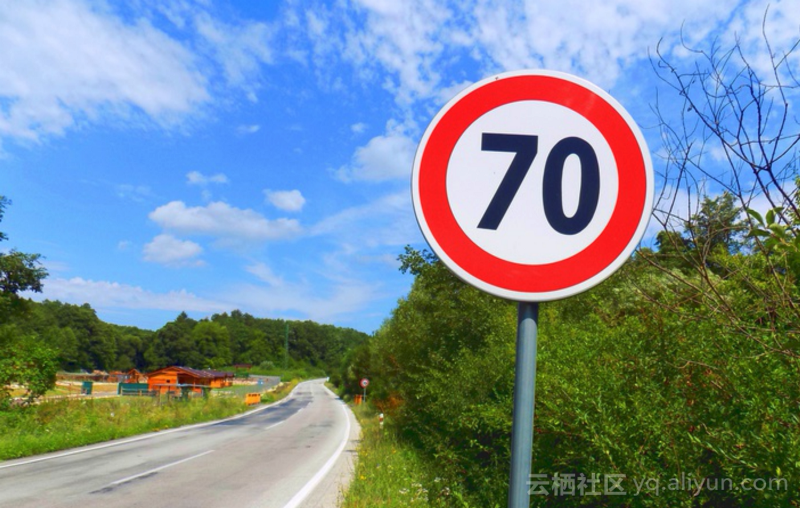# 配置

docker run -it -p 8888:8888 -p 6006:6006 -v ~/traffic:/traffic waleedka/modern-deep-learning

# 准备训练数据BelgiumTSC_Training (171.3MBytes)
BelgiumTSC_Testing (76.5MBytes)

/traffic/datasets/BelgiumTS/Training/
/traffic/datasets/BelgiumTS/Testing/

# 探索数据

def load_data(data_dir):
# Get all subdirectories of data_dir. Each represents a label.
directories = [d for d in os.listdir(data_dir)
if os.path.isdir(os.path.join(data_dir, d))]
# Loop through the label directories and collect the data in
# two lists, labels and images.
labels = []
images = []
for d in directories:
label_dir = os.path.join(data_dir, d)
file_names = [os.path.join(label_dir, f)
for f in os.listdir(label_dir)
if f.endswith(".ppm")]
for f in file_names:
labels.append(int(d))
return images, labels

images, labels = load_data(train_data_dir)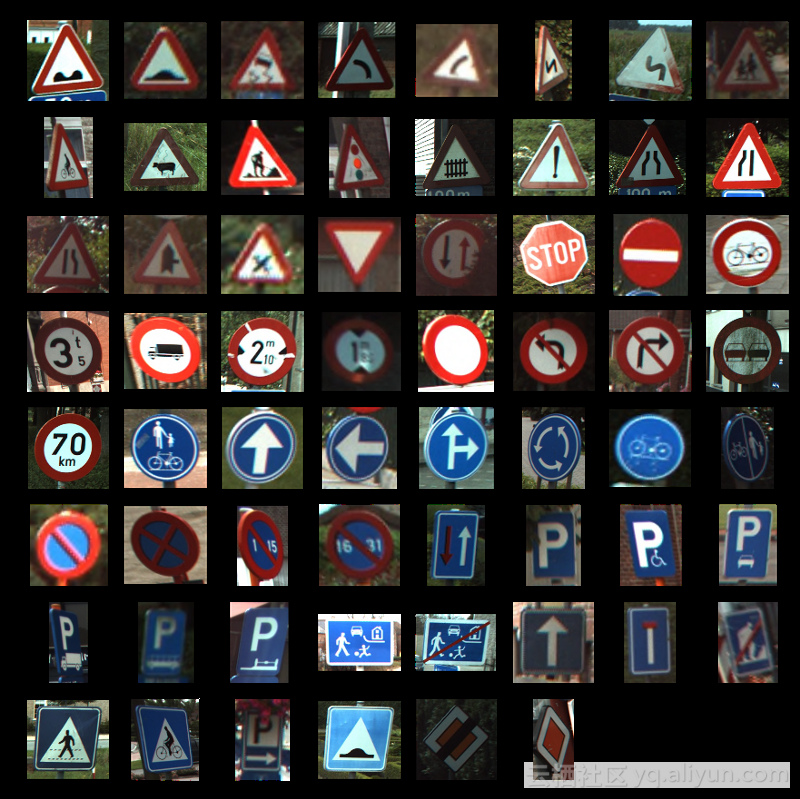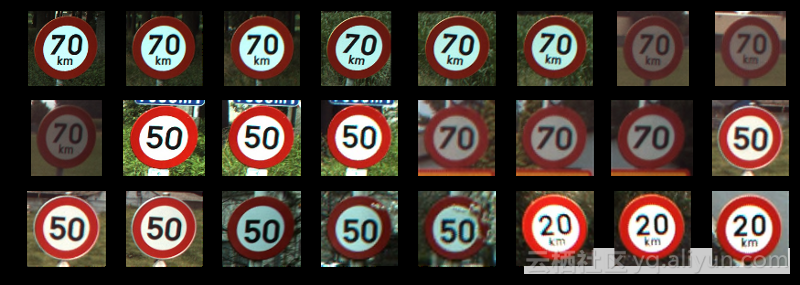# 处理不同大小的图像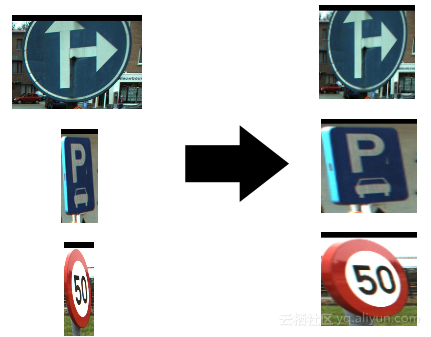for image in images[:5]:
print("shape: {0}, min: {1}, max: {2}".format(
image.shape, image.min(), image.max()))
Output:
shape: (141, 142, 3), min: 0, max: 255
shape: (120, 123, 3), min: 0, max: 255
shape: (105, 107, 3), min: 0, max: 255
shape: (94, 105, 3), min: 7, max: 255
shape: (128, 139, 3), min: 0, max: 255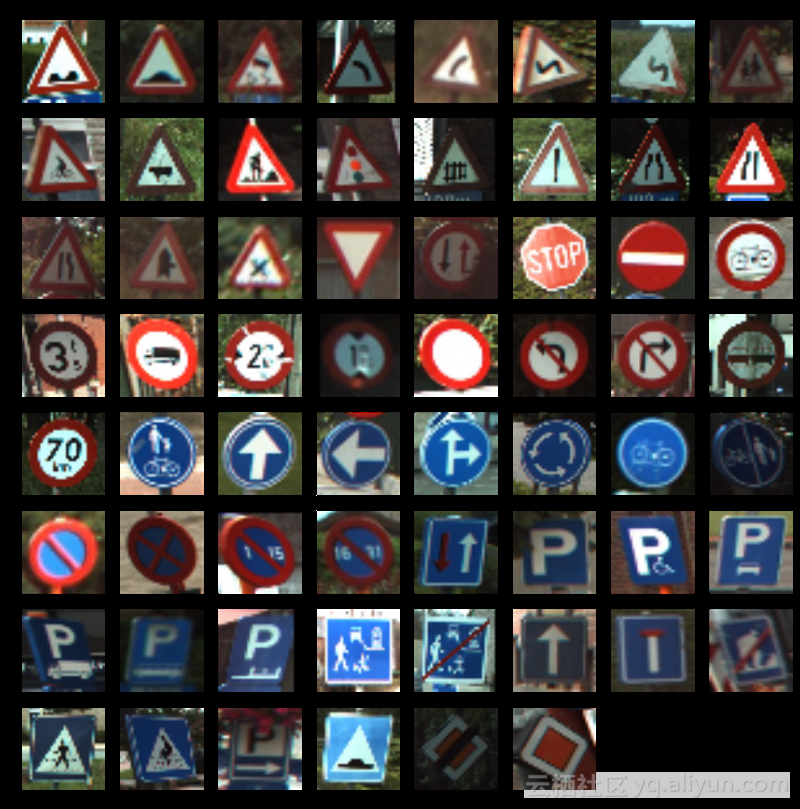# 最小可行模型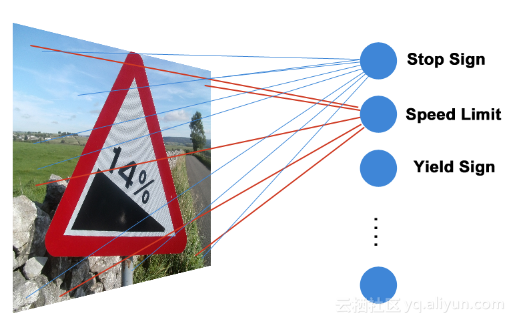y = xW + b

# 构建TensorFlow图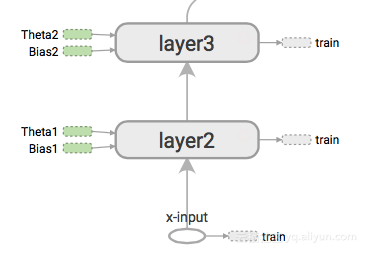TensorFlow用执行图的方式来隐藏神经网络的架构。这个图中包含加、乘、改造等方法。这些操作对数据的张量上进行处理。

with graph.as_default():
# Placeholders for inputs and labels.
images_ph = tf.placeholder(tf.float32, [None, 32, 32, 3])
labels_ph = tf.placeholder(tf.int32, [None])

# Flatten input from: [None, height, width, channels]
# To: [None, height * width * channels] == [None, 3072]
images_flat = tf.contrib.layers.flatten(images_ph)

# Fully connected layer.
# Generates logits of size [None, 62]
logits = tf.contrib.layers.fully_connected(images_flat, 62, tf.nn.relu)

# Convert logits to label indexes (int).
# Shape [None], which is a 1D vector of length == batch_size.
predicted_labels = tf.argmax(logits, 1)

# Define the loss function.
# Cross-entropy is a good choice for classification.
loss = tf.reduce_mean(tf.nn.sparse_softmax_cross_entropy_with_logits(
logits, labels_ph))

# Create training op.

# And, finally, an initialization op to execute before training.
# TODO: rename to tf.global_variables_initializer() on TF 0.12.
init = tf.initialize_all_variables()

graph = tf.Graph()

with graph.as_default():
images_ph = tf.placeholder(tf.float32, [None, 32, 32, 3])
labels_ph = tf.placeholder(tf.int32, [None])

images_ph的占位符形式大概是[None, 32, 32, 3]，这个分别表示[批次，高，宽，通道]，批次是None表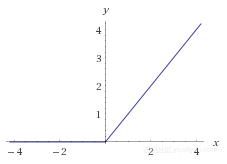f(x) = max(0, x)

# Flatten input from: [None, height, width, channels]
# To: [None, height * width * channels] == [None, 3072]
images_flat = tf.contrib.layers.flatten(images_ph)
# Fully connected layer.
# Generates logits of size [None, 62]
logits = tf.contrib.layers.fully_connected(images_flat, 62,
tf.nn.relu)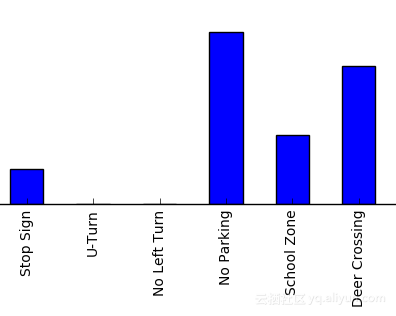logits向量的一行可能是 [0.3, 0, 0, 1.2, 2.1, .01, 0.4, ….., 0, 0]这样的形式。数值越高，说明图片属于标签的可能性越大，logits并不是概率，它的值可以是任意的，而且总和不一定是1。Logists的值是多少无所谓，关键是它们之间相对的大小关系。当然，使用softmax函数也可以将logits转换为概率。

# Convert logits to label indexes.
# Shape [None], which is a 1D vector of length == batch_size.
predicted_labels = tf.argmax(logits, 1)

Argmax的输出是一个0到61的数。

# 损失函数和梯度下降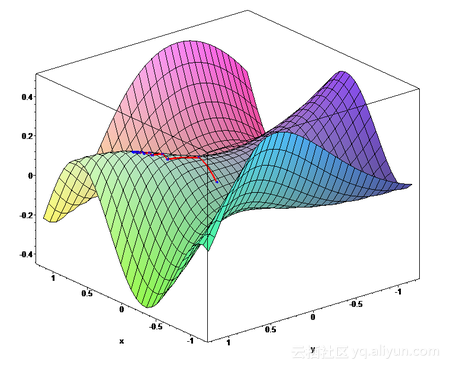loss = tf.reduce_mean(
tf.nn.sparse_softmax_cross_entropy_with_logits(
logits, labels_ph))

train = tf.train.AdamOptimizer(learning_rate=0.001).minimize(loss)

init = tf.initialize_all_variables()

# 训练循环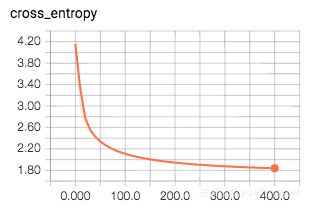session = tf.Session(graph=graph)

session.run(init)

for i in range(201):
_, loss_value = session.run(
[train, loss],
feed_dict={images_ph: images_a, labels_ph: labels_a})
if i % 10 == 0:
print("Loss: ", loss_value)

Loss:  4.2588
Loss:  2.88972
Loss:  2.42234
Loss:  2.20074
Loss:  2.06985
Loss:  1.98126
Loss:  1.91674
Loss:  1.86652
Loss:  1.82595
...

# 使用模型

# Pick 10 random images
sample_indexes = random.sample(range(len(images32)), 10)
sample_images = [images32[i] for i in sample_indexes]
sample_labels = [labels[i] for i in sample_indexes]
# Run the "predicted_labels" op.
predicted = session.run(predicted_labels,
{images_ph: sample_images})
print(sample_labels)
print(predicted)

Output:
[15, 22, 61, 44, 32, 22, 57, 38, 56, 38]
[14  22  61  44  32  22  56  38  56  38]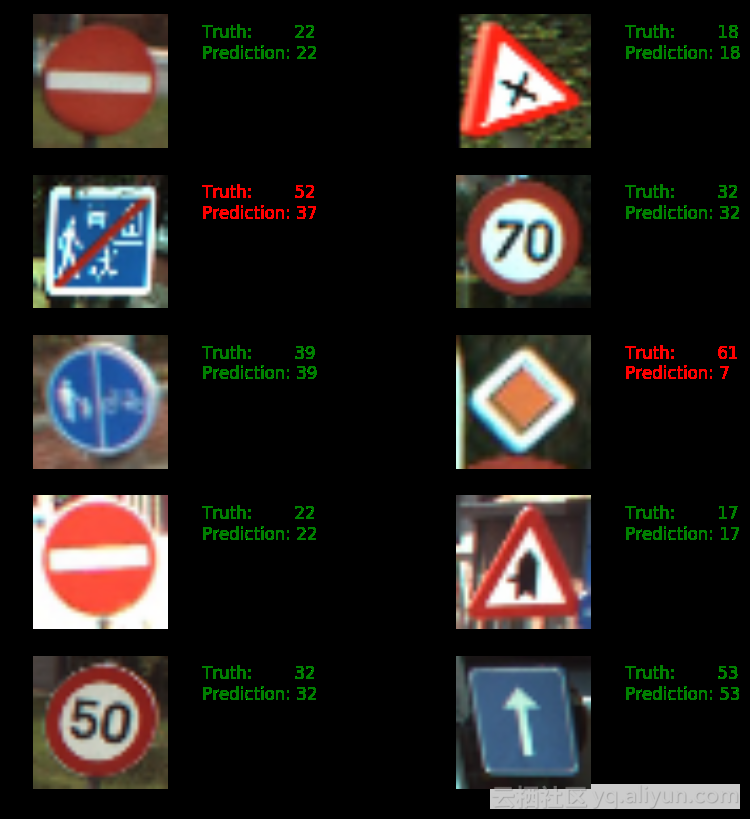# 评估

# Run predictions against the full test set.
predicted = session.run(predicted_labels,
feed_dict={images_ph: test_images32})
# Calculate how many matches we got.
match_count = sum([int(y == y_)
for y, y_ in zip(test_labels, predicted)])
accuracy = match_count / len(test_labels)
print("Accuracy: {:.3f}".format(accuracy))

# 关闭Session

# Close the session. This will destroy the trained model.
session.close()

# 文章原标题《Traffic Sign Recognition with TensorFlow》，作者：Waleed Abdulla，译者：爱小乖

#文章为简译，更为详细的内容，请查看原文DeepMind发布Sonnet 帮你用TensorFlow快速搭建神经网络
841 0TensorFlow 实战卷积神经网络之 LeNet

2872 04398 01216 0TensorFlow实现神经网络入门篇

5884 07735 0911 0Tensorflow系列专题（四）：神经网络篇之前馈神经网络综述
2405 0TensorFlow 卷积神经网络手写数字识别数据集介绍

1461 02024 0
【方向】

696

5

《SaaS模式云原生数据仓库应用场景实践》

《看见新力量：二》电子书